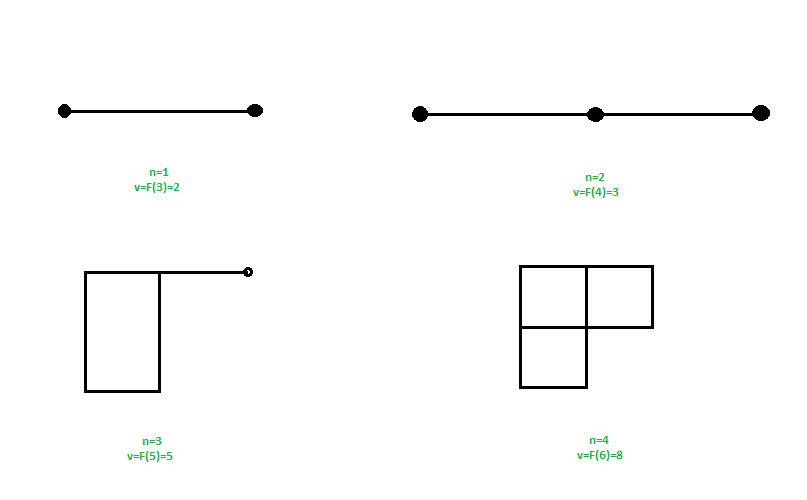# Fibonacci Cube Graph

You are given input as order of graph n (highest number of edges connected to a node), you have to find the number of vertices in a Fibonacci cube graph of order n.

Examples :

```Input : n = 3
Output : 5
Explanation :
Fib(n + 2) = Fib(5) = 5

Input : n = 2
Output : 3
```

## Recommended: Please try your approach on {IDE} first, before moving on to the solution.

A Fibonacci Cube Graph is similar to hypercube graph, but with a fibonacci number of vertices. In fibonacci cube graph only 1 vertex has degree n rest all has degree less than n.
Fibonacci cube graph of order n has F(n + 2) vertices, where F(n) is a n-th fibonacci number,
Fibonacii series : 1, 1, 2, 3, 5, 8, 13, 21, 34……………….For input n as order of graph, find the corresponding fibonacci number at the position n + 2.
where F(n) = F(n – 1) + F(n – 2)

Approach : Find the (n + 2)-th fibonacci number.

Below is the implementation of above approach :

## C++

 `// CPP code to find vertices in a fibonacci ` `// cube graph of order n ` `#include ` `using` `namespace` `std; ` ` `  `// function to find fibonacci number ` `int` `fib(``int` `n) ` `{ ` `    ``if` `(n <= 1) ` `        ``return` `n; ` `    ``return` `fib(n - 1) + fib(n - 2); ` `} ` ` `  `// function for finding number of vertices  ` `// in fibonacci cube graph ` `int` `findVertices (``int` `n) ` `{ ` `    ``// return fibonacci number for f(n + 2)  ` `    ``return` `fib(n + 2); ` `} ` ` `  `// driver program ` `int` `main() ` `{ ` `    ``// n is the order of the graph ` `    ``int` `n = 3; ` `    ``cout << findVertices(n); ` `    ``return` `0; ` `} `

## Java

 `// java code to find vertices in a fibonacci ` `// cube graph of order n ` `public` `class` `GFG { ` `     `  `    ``// function to find fibonacci number ` `    ``static` `int` `fib(``int` `n) ` `    ``{ ` `        ``if` `(n <= ``1``) ` `            ``return` `n; ` `        ``return` `fib(n - ``1``) + fib(n - ``2``); ` `    ``} ` `     `  `    ``// function for finding number of vertices  ` `    ``// in fibonacci cube graph ` `    ``static` `int` `findVertices (``int` `n) ` `    ``{ ` `        ``// return fibonacci number for f(n + 2)  ` `        ``return` `fib(n + ``2``); ` `    ``} ` `         `  `    ``public` `static` `void` `main(String args[]) { ` `         `  `        ``// n is the order of the graph ` `        ``int` `n = ``3``; ` `        ``System.out.println(findVertices(n)); ` `    ``} ` `} ` ` `  `// This code is contributed by Sam007 `

## Python3

 `# Python3 code to find vertices in  ` `# a fibonacci cube graph of order n  ` ` `  `# Function to find fibonacci number  ` `def` `fib(n):  ` ` `  `    ``if` `n <``=` `1``:  ` `        ``return` `n ` `         `  `    ``return` `fib(n ``-` `1``) ``+` `fib(n ``-` `2``)  ` ` `  `# Function for finding number of  ` `# vertices in fibonacci cube graph  ` `def` `findVertices(n):  ` ` `  `    ``# return fibonacci number  ` `    ``# for f(n + 2)  ` `    ``return` `fib(n ``+` `2``)  ` ` `  `# Driver Code ` `if` `__name__ ``=``=` `"__main__"``:  ` ` `  `    ``# n is the order of the graph  ` `    ``n ``=` `3` `    ``print``(findVertices(n))  ` ` `  `# This code is contributed  ` `# by Rituraj Jain `

## C#

 `// C# code to find vertices in a fibonacci ` `// cube graph of order n ` `using` `System; ` ` `  `class` `GFG { ` `     `  `    ``// function to find fibonacci number ` `    ``static` `int` `fib(``int` `n) ` `    ``{ ` `        ``if` `(n <= 1) ` `            ``return` `n; ` `        ``return` `fib(n - 1) + fib(n - 2); ` `    ``} ` `     `  `    ``// function for finding number of  ` `    ``// vertices in fibonacci cube graph ` `    ``static` `int` `findVertices (``int` `n) ` `    ``{ ` `         `  `        ``// return fibonacci number for ` `        ``// f(n + 2)  ` `        ``return` `fib(n + 2); ` `    ``} ` `     `  `    ``// Driver code ` `    ``static` `void` `Main() ` `    ``{ ` `         `  `        ``// n is the order of the graph ` `        ``int` `n = 3; ` `         `  `        ``Console.Write(findVertices(n)); ` `    ``} ` `} ` ` `  `// This code is contributed by Sam007 `

## PHP

 ` `

Output :

```5
```

Note that the above code can be optimized to work in O(Log n) using efficient implementations discussed in Program for Fibonacci numbers

Attention reader! Don’t stop learning now. Get hold of all the important DSA concepts with the DSA Self Paced Course at a student-friendly price and become industry ready.

My Personal Notes arrow_drop_upCheck out this Author's contributed articles.

If you like GeeksforGeeks and would like to contribute, you can also write an article using contribute.geeksforgeeks.org or mail your article to contribute@geeksforgeeks.org. See your article appearing on the GeeksforGeeks main page and help other Geeks.

Please Improve this article if you find anything incorrect by clicking on the "Improve Article" button below.

Improved By : Sam007, rituraj_jain

Article Tags :
Practice Tags :

Be the First to upvote.

Please write to us at contribute@geeksforgeeks.org to report any issue with the above content.